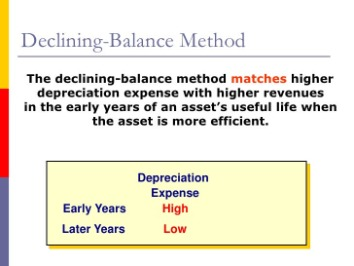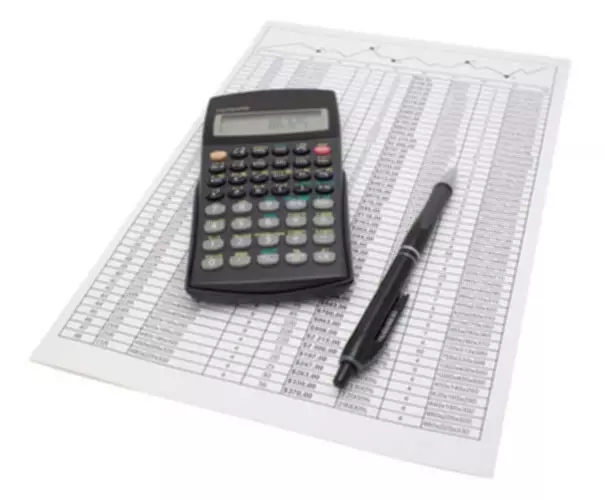Select PageOnce you fully depreciate the asset’s value, you have to record the salvage value of the asset and close the account. However, a new business brings the responsibility of managing the fundamental and technical aspects of marketing, finance, etc. You will have to learn about the intricacies and functioning of these key divisions that will drive your company’s growth.

• We can understand how the depreciation expense is calculated each year under the double-declining method from the below schedule.
• While you don’t calculate salvage value up front when calculating the double declining depreciation rate, you will need to know what it is, since assets are depreciated until they reach their salvage value.
• If you use the double-declining balance method to calculate the value of depreciation, the value would be high in the initial years.
• Not all assets are purchased conveniently at the beginning of the accounting year, which can make the calculation of depreciation more complicated.
• Similarly, compared to the standard declining balance method, the double-declining method depreciates assets twice as quickly.

Then, we need to calculate the depreciation rate, which is explained under the next heading. In the next step, we need to multiply the beginning book value by twice the depreciation rate and deduct the depreciation expense from the beginning value to arrive at the remaining value. A similar process will be repeated each year throughout the asset’s useful life, or till the point we reach the salvage value of the asset. Double declining balance depreciation is an accelerated depreciation method.

## Alternative Methods

The most basic type of depreciation is the straight line depreciation method. You use it to write off the same depreciation expense every year. So, if an asset cost \$1,000, you might write off \$100 every year for 10 years. Depreciation rates used in the declining balance method could be 150%, 200% , or 250% of the straight-line rate. When the depreciation rate for the declining balance method is set as a multiple, doubling the straight-line rate, the declining balance method is effectively the double-declining balance method. Over the depreciation process, the double depreciation rate remains constant and is applied to the reducing book value each depreciation period. The book value, or depreciation base, of an asset, declines over time.

### What is the declining balance method of assets depreciation?

Under the declining balance method, depreciation is charged on the book value of the asset and the amount of depreciation decreases every year.

The company is less profitable in the early years than in later years; thus, it will be difficult to measure its true operational profitability. Cash And Cash EquivalentsCash and Cash Equivalents are assets that are short-term and highly liquid investments that can be readily converted into cash and have a low risk of price fluctuation. Cash and paper money, US Treasury bills, undeposited receipts, and Money Market funds are its examples. They are normally found https://www.bookstime.com/ as a line item on the top of the balance sheet asset. Multiply the beginning period book value by twice the depreciation rate to find the depreciation expense. Using the steps outlined above, let’s walk through an example of how to build a table that calculates the full depreciation schedule over the life of the asset. Depreciation is an accounting method of allocating the cost of a tangible asset over its useful life to account for declines in value over time.

## Double-Declining Balance (DDB) Depreciation Formula

Then record the depreciation journal entry in Year 2 as a debit of \$7,380 to the Depreciation Expense-Business Truck account and a credit of \$7,380 to the Accumulated Depreciation-Business Truck account. Then record the depreciation journal entry in Year 1 as a debit of \$9,000 to the Depreciation Expense-Business Truck account and a credit of \$9,000 to the Accumulated Depreciation-Business Truck account.

Depreciable property is an asset that is eligible for depreciation treatment in accordance with IRS rules. These are provided by the IRS and vary by value and type of asset.

## What is the double declining balance method of depreciation?

Firms want to use the double declining balance method because the net income of the asset in its early years is lower than it would have been by using the straight-line method. When you file your taxes, you have to predict your annual income. If you use the double-declining balance method, the book value of the assets will change every year. The changing values can affect your business forecasting function, and you might find it challenging to come to a fair prediction. The first step to understanding what is depreciation method you should use for your business is knowing what depreciation is.Use this calculator to calculate the accelerated depreciation by Double Declining Balance Method or 200% depreciation. For other factors besides double use the Declining Balance Method Depreciation Calculator. Salvage Value → The residual value of the fixed asset at the end of its useful life – most companies assume this to be zero. Useful Life Assumption → The useful life assumption is the implied number of years in which a fixed asset is assumed to provide economic benefits to the company. Once you’ve done this, you’ll have your basic yearly write-off. Formula, the depreciation rate remains the same and is applied to the ending value of the last year. Salvage value is the estimated book value of an asset after depreciation.

## How Small Business Owners Can Cope with Inflation and a Possible Recession

Because of the high number of miles you expect to put on the truck, you estimate its useful life at five years. At the beginning of the second year, the fixture’s book value will be \$80,000, which is the cost of \$100,000 minus the accumulated depreciation of \$20,000.

• Accumulated DepreciationThe accumulated depreciation of an asset is the amount of cumulative depreciation charged on the asset from its purchase date until the reporting date.
• It is an accelerated depreciation method that depreciates the asset value at twice the rate in comparison to the depreciation rate used in the straight-line method.
• Then record the depreciation journal entry in year 9 as a debit of \$2,488.09 to the Depreciation Expense-Business Truck account and a credit of \$2,488.09 to the Accumulated Depreciation-Business Truck account.
• However, there are certain advantages to accelerated depreciation methods.

Instead, compute the difference between the beginning book value and salvage value to compute the depreciation expense. Eric is a staff writer at Fit double declining balance method Small Business and CPA focusing on accounting content. He spends most of his time researching and studying to give the best answer to everyone.

## How is Declining Balance Depreciation Used?

With your second year of depreciation totaling \$6,720, that leaves a book value of \$10,080, which will be used when calculating your third year of depreciation. The following table illustrates double declining depreciation totals for the truck. The next chart displays the differences between straight line and double declining balance depreciation, with the first two years of depreciation significantly higher. The “double” or “200%” means two times straight-line rate of depreciation.

• The DDB depreciation method is easy to implement and track in most accounting software.
• It is a contra-account, the difference between the asset’s purchase price and its carrying value on the balance sheet.
• The double-declining balance method depreciates or reduces the asset’s value twice as fast as the other methods.
• To depreciate the entire value of the asset, you will eventually have to switch to the straight-line method for calculating depreciation.